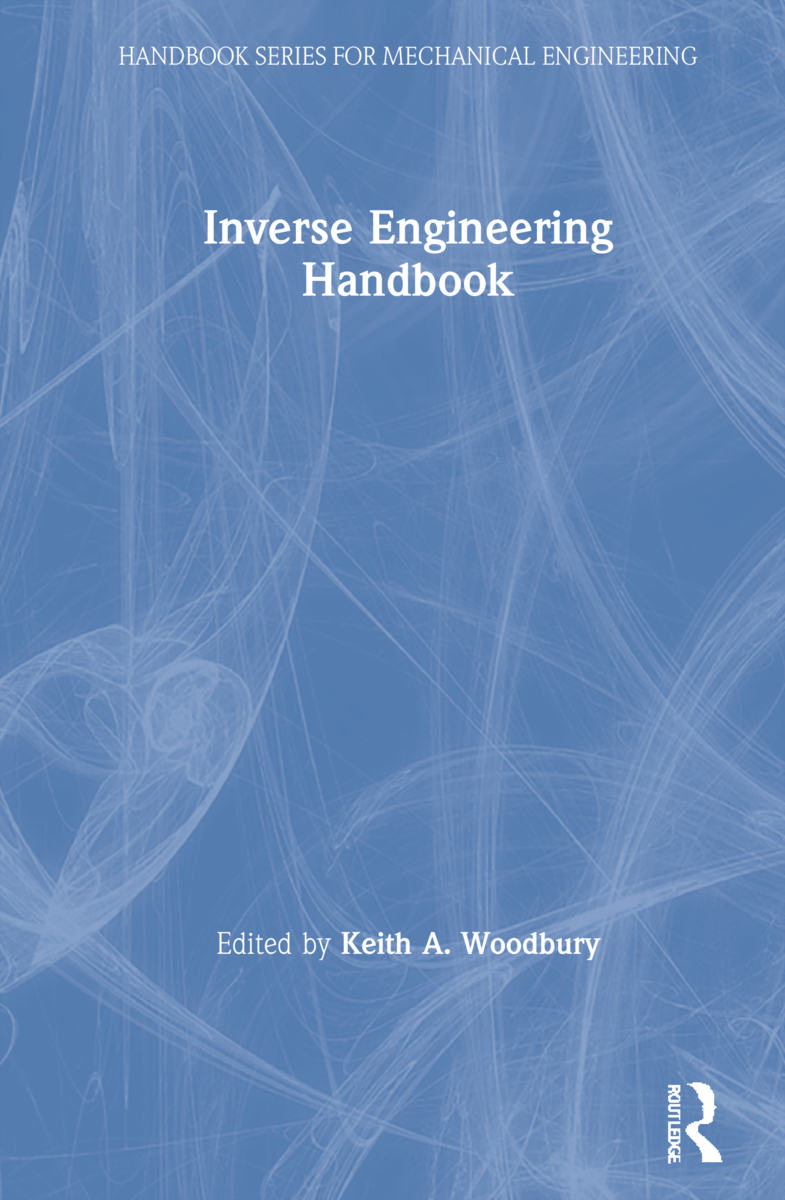Inverse Engineering Handbook

1st Edition

CRC Press

480 pages | 184 B/W Illus.

Purchasing Options:\$ = USD
Hardback: 9780849308611
pub: 2002-09-25
SAVE ~\$55.00
\$275.00
\$220.00
x
eBook (VitalSource) : 9780429117893
pub: 2002-09-25
from \$137.50

FREE Standard Shipping!

Description

Inverse problems have been the focus of a growing number of research efforts over the last 40 years-and rightly so. The ability to determine a "cause" from an observed "effect" is a powerful one. Researchers now have at their disposal a variety of techniques for solving inverse problems, techniques that go well beyond those useful for relatively simple parameter estimation problems. The question is, where can one find a single, comprehensive resource that details these methods?

The answer is the Inverse Engineering Handbook. Leading experts in inverse problems have joined forces to produce the definitive reference that allows readers to understand, implement, and benefit from a variety of problem-solving techniques. Each chapter details a method developed or refined by its contributor, who provides clear explanations, examples, and in many cases, software algorithms. The presentation begins with methods for parameter estimation, which build a bridge to boundary function estimation problems. The techniques addressed include sequential function estimation, mollification, space marching techniques, and adjoint, Monte Carlo, and gradient-based methods. Discussions also cover important experimental aspects, including experiment design and the effects of uncertain parameters.

While many of the examples presented focus on heat transfer, the techniques discussed are applicable to a wide range of inverse problems. Anyone interested in inverse problems, regardless of their specialty, will find the Inverse Engineering Handbook to be a unique and invaluable compendium of up-to-date techniques.

Reviews

"…serves as a good introduction and tutorial for this important area of applied mathematics. Several of the articles provide extensive MATLAB codes for specific problems."

--James E. Epperson, Mathematical Reviews, 2004

SEQUENTIAL METHODS IN PARAMETER ESTIMATION

James V. Beck, Professor Emeritus, Michigan State University, USA

Abstract

Introduction

Parameter vs. Function Estimation

Common Research Paradigms in Heat Transfer

Sequential Estimation over Experiments for Linear Problems

Ill-Posed Problems: Tikhonov Regularization

Matrix Form of Taylor Series Expansion

Gauss Method of Minimization for Nonlinear Estimation Problems

Confidence Regions

Optimal Experiments

Summary

References

SEQUENTIAL FUNCTION SPECIFICATION METHOD USING FUTURE TIMES FOR FUNCTION ESTIMATION

Keith A. Woodbury, The University of Alabama, USA

Abstract

Nomenclature

Introduction

Linear Problems

Nonlinear Problems

Summary

References

THE ADJOINT METHOD TO COMPUTE THE NUMERICAL SOLUTIONS OF INVERSE PROBLEMS

Yvon Jarny, Ecole Polytechnique de L'Université de Nantes, France

Introduction

Modelling Equations

Least Squares and Gradient Algorithms

Lagrange Multipliers

The Adjoint Method to Minimize the LS-Criterion with Algebraic Modelling Equations

The Adjoint Method to Minimize the LS-Criterion with Integral Modelling Equation

Adjoint Method to Minimize LS-Criteria with Ordinary Differential Equations as Constraints

Adjoint Method to Minimize LS-Criteria with Partial Differential Equations as Constraints

Conclusion and Summary

References

MOLLIFICATION AND SPACE MARCHING

Diego A. Murio, University of Cincinnati, USA

Mollification In R1

Data Smoothing

Identification of Parameters in 1-D IHCP

Discrete Mollification in R2

References

INVERSE HEAT CONDUCTION USING MONTE CARLO METHOD

A. Haji-Sheikh, University of Texas at Arlington, USA

Introduction

Introduction to Monte Carlo Method

Random Walks in Direct Monte Carlo Simulation

Monte Carlo Method for Inverse Heat Conduction

Conclusion

Nomenclature

References

CORRELATED DATA AND STOCHASTIC PROCESSES

Ashley Emery, University of Washington, USA

Introduction

Correlation and Its Effect on Precision

Least Squares Estimation and Linearization

Determination of

Ergodic and Stationary Processes

Uncertain Parameters

Bayesian Probabilities, Prior Information, and Uncertain Parameters

Conclusions

OPTIMAL EXPERIMENT DESIGN

Aleksey V. Nenarokomov, Moscow State Aviation Institute, Russia

Introduction

Brief Historical Analysis of Background and Survey

Experiment Design Problem Statement

Iterative Method of Optimal Design of Thermosensors Installation and Time of Signals Readings

Experiment Design for Lumped Parameter Systems

Conclusions

References

BOUNDARY ELEMENT TECHNIQUES FOR INVERSE PROBLEMS

Thomas J. Martin, Pratt & Whitney Engine Company and George S. Dulikravich, University of Texas at Arlington, USA

Introduction

Inverse Heat Conduction

Ill-Posed Boundary Conditions in Fluid Flow

Ill-Posed Surface Tractions and Deformations in Elastostatics

Inverse Detection of Sources

Transient Problems

References

Woodbury\, Keith A.